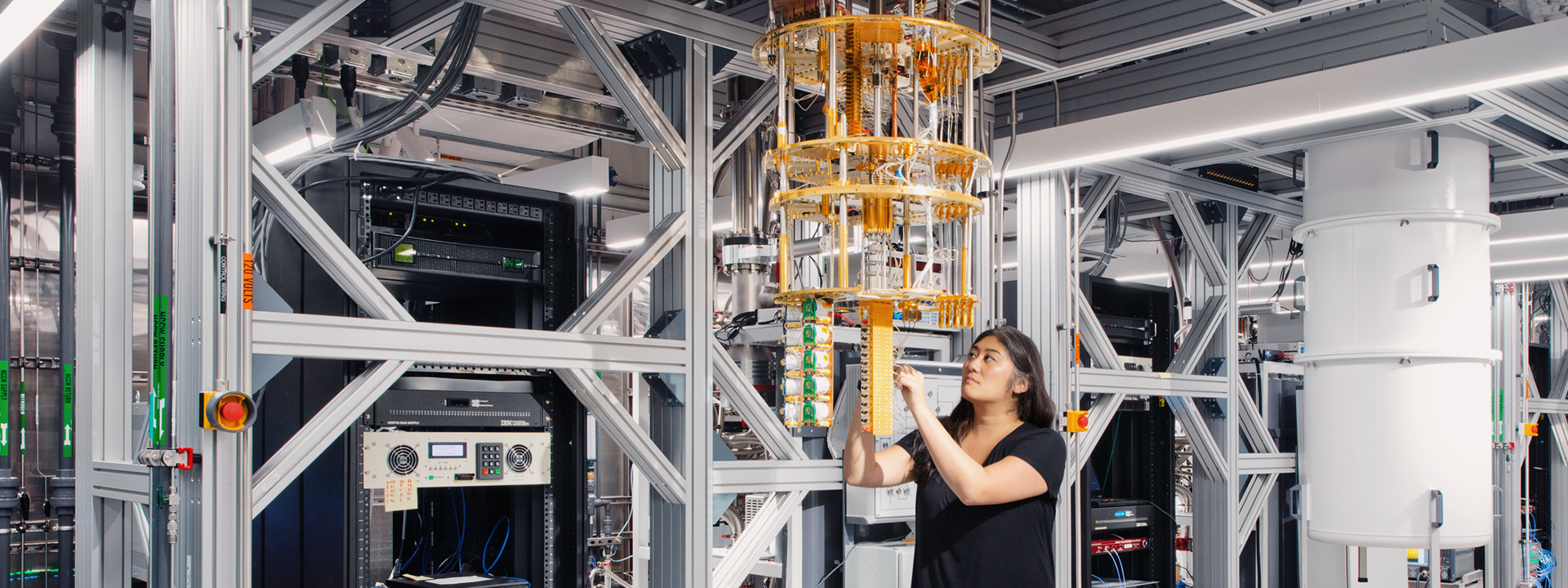# IBM Runs Largest-Ever Quantum Program

Researchers use mathematical error correction to target correct resultsIBM is researching quantum computers that can correct their own errors. IBM

IBM researchers have run the largest quantum program yet, using an enhanced 127-qubit quantum circuit with the ability to conduct 1,700 individual operations. They also learned how to mitigate the errors that come with larger qubit circuits.

Previously, IBM could only deploy a 26-qubit quantum circuit that performed 1,080 individual operations. The researchers developed the new circuit to calculate what would happen if they forced a chain of electron-like particles to interact with each other. Since classical computers could not fully resolve this problem, the team created superconducting qubits that ran programs based on microwave pulse sequences.

By adjusting these microwave pulses, the researchers learned how to repeat and multiply qubit errors computationally. This exercise enabled them to mathematically reverse qubit errors in the same manner.

Qubit Error Mitigation

Qubits exercise what is known as quantum superposition, acting as if they are in two states at once. Unlike a computer

bit that can be either 0 or 1, a qubit can effectively be in 0 and 1 and positions in between. As a result, the more qubits a computer uses, the more complex calculations it can run.

However, adding more qubits also produces more error-filled answers. These errors reflect the inherent sensitivity of quantum computing to noise. Given enough qubits, failure to remove excess error-filled answers will yield unhelpful results.

In addition, eliminating these errors has proven problematic, as many of them originate from computer hardware. Rather than eliminate these error-filled answers, however, the IBM team sought to convert them into clear answers. They came to this solution by repeating and multiplying these errors, thus learning how to correct the error away mathematically. Once the IBM researchers mathematically reversed an error, they then sought to produce quantum calculations without any errors.

Researchers running large qubit operations previously had to approximate the results of a quantum calculation. With this new method, researchers can run large qubit operations with the same expectation they would have with a classical computer. In the future, researchers aim to create quantum computers that correct errors as they go.